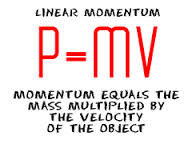# Difference between Impulse and Momentum

##### Key Difference: Impulse can be described as a quantity that states an effect of a net force acting on an object. Momentum is described as a quantity that states an object’s resistance to stopping. Impulse and momentum are directly related to each other. Impulse can also be described as a change in momentum.Impulse can be described as a quantity that states an effect of a net force acting on an object. It is defined as a product of force and time. Impulse can also be stated as the integral of force acting on an object with respect to time. It basically changes the momentum of an object. Impulse can be easily understood by an example - a hammer strike defines a large force that acts for a very short period of time. Impulse is expressed in Kgm/s and Impulsive force is expressed in Newton (N).Momentum is described as a quantity that states an object’s resistance to stopping. Therefore, it can also be defined as the “Inertia in Motion.” Momentum is possessed by any type of entity that is moving. Linear momentum is a product of mass and velocity. It is hard to stop a heavy vehicle in comparison to a light vehicle. This is due to the fact that the momentum of a heavy car is greater than a light vehicle, because of the car's mass. Impulse and momentum are vector quantities as both are products of a scalar and a vector quantity. Newton’s second law of motion is expressed as - the rate of change of momentum is proportional to the net force and is in the direction of that force.

Comparison between Impulse and Momentum:

 Impulse Momentum Definition Impulse can be described as a quantity that states an effect of a net force acting on an object. Momentum is described as a quantity that states an object’s resistance to stopping. Impulse and momentum are directly related to each other. Symbol J (boldface) P (boldface) Formula Product of the average net force acting on and object. J = F Δt Product of an object’s mass and velocity. p = m v Quantity Vector Vector S.I. Unit N s Newton second kg m/s Kilogram meter per second Zero value If the force is non-constant, and reverses itself over time, it can give a zero impulse. A body has no momentum, if it is in rest.

Image Courtesy: batesville.k12.in.us, physics4kids.com